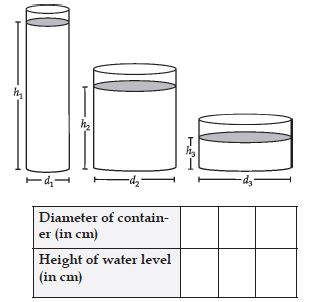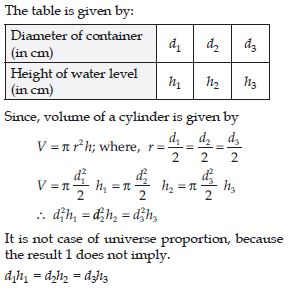# NCERT Solutions for Class 8 Math Chapter 13 - Direct and Inverse Proportions

##### Question 1:

Observe the following tables and find if x and y are directly proportional: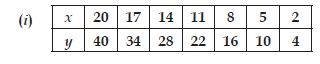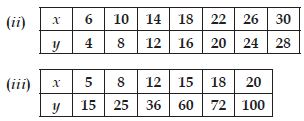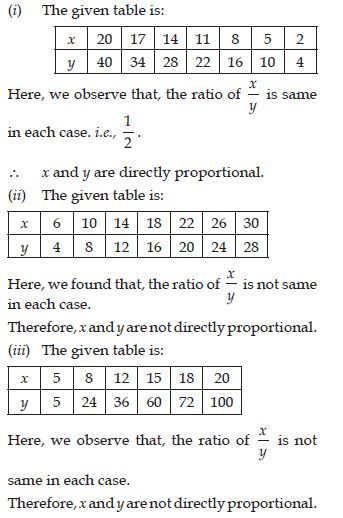##### Question 2:

Principal = ₹1000, Rate = 8% per annum.
Fill in the following table and find which type of interest (simple or compound) changes in direct proportion with time period.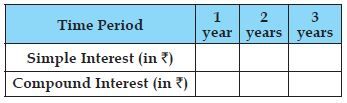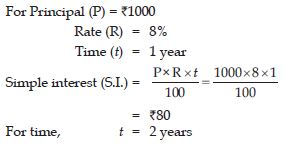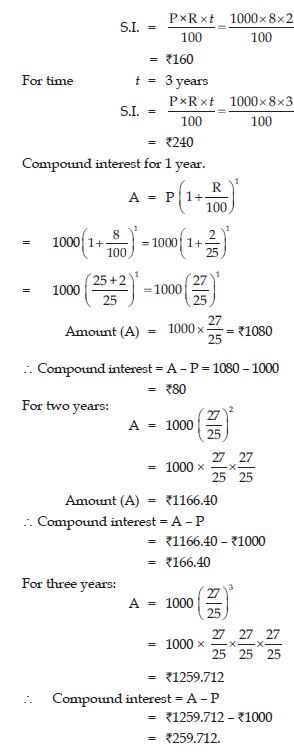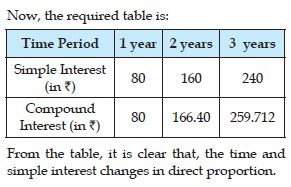##### Question 3:

If we fix time period and the rate of interest, simple interest changes proportionally with principle. Would there be a similar relationship for compound interest? Why?

Since, the principal amount for compound interest is not same every year, so, the compound interest does not change proportionally for a fixed time period and rate of interest. There cannot be a similar relationship for simple interest.

##### Question 4:

Take a map of your state. Note the scale used there using a ruler, measure the “map distance” between any two cities, calculate the actual distance between them.

Let the distance between any two cities be 2 cm.
[where scale: 1 cm = 400 km]
∴ Actual distance between them
= 2 × 400 km = 800 km

##### Question 5:

Following are the car parking charges near a railway station upto:
4 hours ₹60
8 hours ₹100
12 hours ₹140
24 hours ₹180
Check if the parking charges are in direct proportion to the parking time.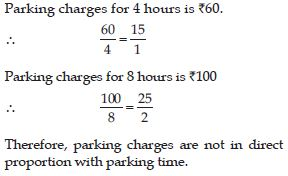##### Question 6:

A mixture of paint is prepared by mixing 1 part of red pigments with 8 parts of base.
In the following table, find the parts of base that need to be added.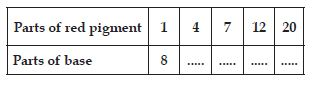The given table is: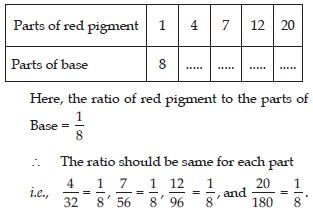##### Question 7:

If 1 part of a red pigment requires 75 mL of base, how much red pigment should we mix with 1800 mL of base?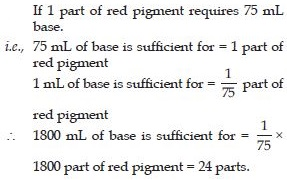##### Question 8:

A machine in a soft drink factory fills 840 bottles in six hours. How many bottles will it fill in five hours?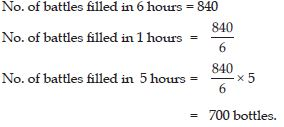##### Question 9:

A photograph of a bacteria enlarged 50,000 times attains a length of 5 cm. What is the actual length of the bacteria? If the photograph is enlarged 20,000 times only, what would be its enlarged length?

Length of bacteria when enlarged 50,000 times = 5 cm Length of bacteria when enlarged 1 time (i.e., actual position)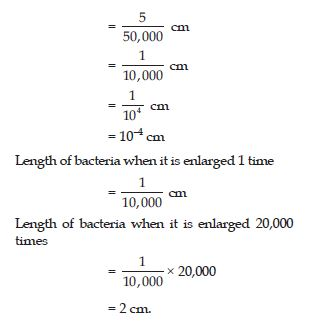##### Question 10:

In a model of a ship, the mast is 9 cm high, while the mast of the actual ship is 12 m high. If the length of the ship is 28 m, how long is the model ship?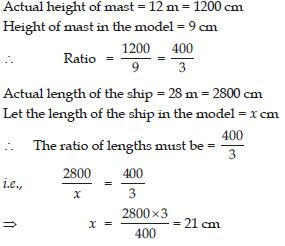##### Question 11:

Suppose 2 kg of sugar contains 9 × 106 crystals. How many sugar crystals are there in 5 kg of sugar?

No. of sugar crystals in 2 kg of sugar = 9 × 106
No. of sugar crystals in 1 kg of sugar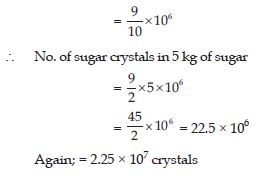##### Question 12:

Suppose 2 kg of sugar contains 9 × 106 crystals. How many sugar crystals are there in 1.2 kg of sugar?

No. of sugar crystals in 2 kg of sugar = 9 × 106
No. of sugar crystals in 1 kg of sugar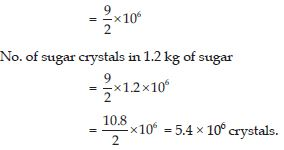##### Question 13:

Rashmi has a road map with a scale of 1 cm representing 18 km. She drives on a road for 72 km. What would be her distance covered in the map?

18 km on road represents 1 cm on map.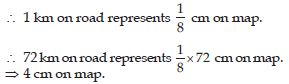##### Question 14:

A 5 m 60 cm high vertical pole casts a shadow 3 m 20 cm long. Find at the same time the length of the shadow cast by another pole 10 m 50 cm high.

If a pole of height 5 m 60 cm i.e., (560 cm) casts a shadow = 3 m 20 cm = 320 cm
Then, a pole of height 1 cm casts a shadow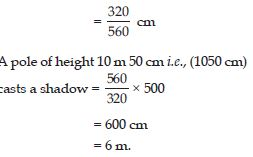##### Question 15:

A 5 m 60 cm high vertical pole casts a shadow 3 m 20 cm long. Find at the same time the height of a pole which casts a shadow of 5 m long.

If shadow of length 3 m 20 cm i.e., 320 cm
is cast by a pole = 560 cm long
If shadow of length 1 cm i.e., 320 cm is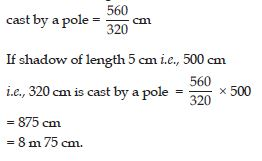##### Question 16:

A loaded truck travels 14 km in 25 minutes. If the speed remains the same, how far can it travel in 5 hours?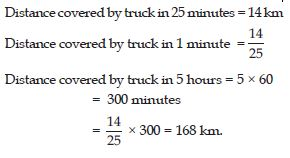##### Question 17:

On a squared paper, draw five square of different sides. Write the following information in a tabular form.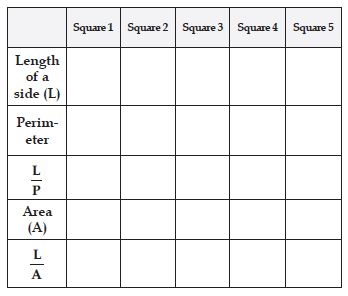Find whether the length of a side is in direct proportion to:
(a) The perimeter of the square.
(b) The area of the square.

From the table, we observe that
(a) Length of a side is in direct proportion to the perimeter of the square.
(b) Length of a side is not in direct proportion to the area of the square.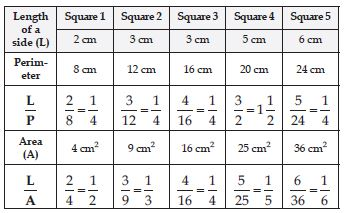##### Question 18:

The following ingredients are required to make halwa for 5 persons Suji/Rawa = 250 g, sugar = 300 g, ghee = 200 g, water = 500 mL.
Using the concept of proportion, estimate the changes in the quantity of ingredients, to prepare halwa for your class.

Suppose there are 30 students in the class.
The ingredients provided is for 5 persons. So, we need the take the multiple of 6 for each ingredients to prepare halwa.

##### Question 19:

Take a square paper and arrange 48 counters on it in different number of rows as shown below.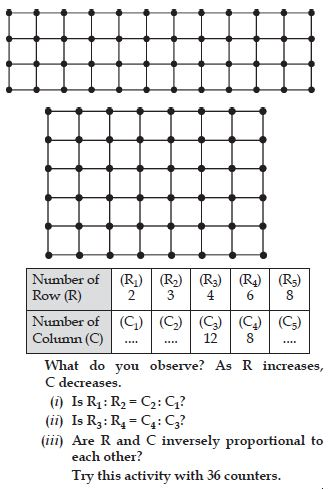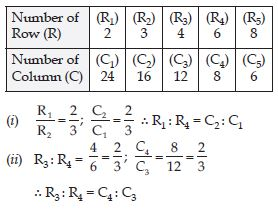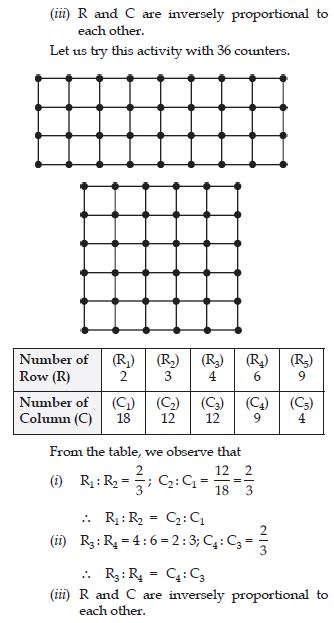##### Question 20:

Observe the following tables and find which pair of variables (here x and y) are in inverse proportion.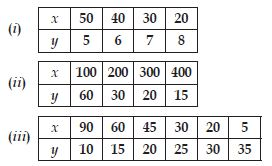(i) In table given here, the product xy is not constant.
x and y are not in inverse proportion.
(ii) In table given here, the product xy is constant.
i.e., 6000.
∴ x and y are in inverse proportion.
(iii) In table given here, the product xy is not constant.
∴ x and y are not in inverse proportion.

##### Question 21:

Which of the following are in inverse proportion?
(i) The number of workers on a job and the time to complete the job.
(ii) The time taken for a journey and the distance travelled in a uniform speed.
(iii) Area of cultivated land and the crop harvested.
(iv) The time taken for a fixed journey and the speed of the vehicle.
(v) The population of a country and the area of land per person.

(i), (iv) and (v) statements are in inverse proportion.

##### Question 22:

In a Television game show, the prize money of ₹1,00,000 is to be divided equally amongst the winners. Complete the following table and find whether the prize money given to an individual winner is directly or inversely proportional to the number of winners?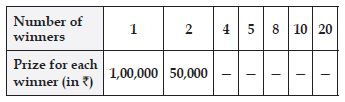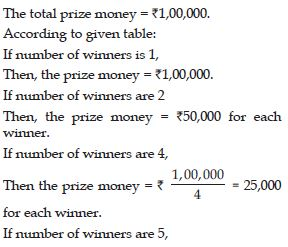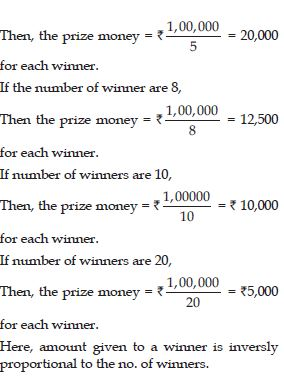##### Question 23:

Rehman is making a wheel using spokes.
He wants to fix equal spokes in such a way that the angles between any pair of consecutive spokes are equal. Help him by completing the following table: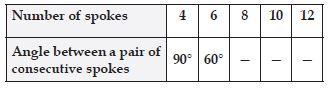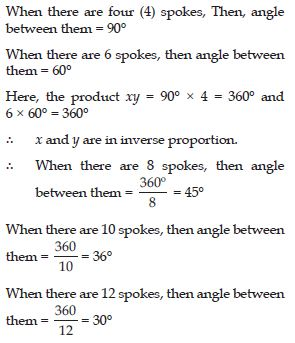##### Question 24:

Rehman is making a wheel using spokes.
He wants to fix equal spokes in such a way that the angles between any pair of consecutive spokes are equal. Help him by completing the following table:Are the number of spokes and the angles formed between the pairs of consecutive spokes in inverse proportion?Yes.

##### Question 25:

Rehman is making a wheel using spokes.
He wants to fix equal spokes in such a way that the angles between any pair of consecutive spokes are equal. Help him by completing the following table:Calculate the angle between a pair of consecutive spokes on a wheel with 15 spokes.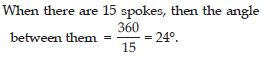##### Question 26:

Rehman is making a wheel using spokes.
He wants to fix equal spokes in such a way that the angles between any pair of consecutive spokes are equal. Help him by completing the following table:How many spokes would be needed, if the angle between a pair of consecutive spokes is 40°?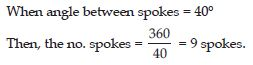##### Question 27:

If a box of sweets is divided among 24 children, they will get 5 sweets each. How many would each get, if the number of the children is reduced by 4?

No. of children = 24
No. of sweets that each children get = 5
∴ Total no. of sweets = 24 × 5 = 120
When no. of children = 20
(i.e., reduced by 4)
∴ No. of sweets that each children get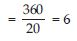##### Question 28:

A farmer has enough food to feed 20 animals in his cattle for 6 days. How long would the food last if there were 10 more animals in his cattle?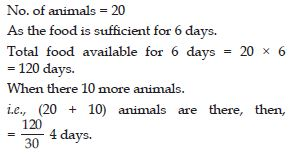##### Question 29:

A contractor estimates that 3 persons could rewire Jasminder’s house in 4 days. If he uses 4 persons instead of three, how long should they take to complete the job?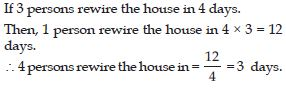##### Question 30:

A batch of bottles were packed in 25 boxes with 12 bottles in each box. If the same batch is packed using 20 bottles in each box, how many boxes would be filled?

No. of bottles in 1 box = 12
No. of bottles in 25 boxes = 12 × 25 = 300 bottles.
When, 20 bottles one in each box, then the no.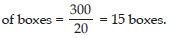##### Question 31:

A factory requires 42 machines to produce a given number of articles in 63 days. How many machines would be required to produce the same number of articles in 54 days?

42 machines can produce given no. of articles in 63 days.
1 machine can produce given no. of articles = 63 × 42 days.
Now, to produce same no. of articles is 54 days,##### Question 32:

A car takes 2 hours to reach a destination by travelling at the speed of 60 km/h. How long will it take when the car travels at the speed of 80 km/h?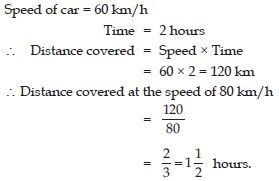##### Question 33:

Two persons could fit new windows in a house in 3 days.
(i) One of the persons fell ill before the work started. How long would the job take now?
(ii) How many persons would be needed to fit the windows in one day?

No. of persons = 2
(i) No. of days required to fit windows = 3
∴ 1 person can fit the windows in = 3 × 2 = 6 days
(ii) No. of persons required to fit the windows in 1 day = 2 × 3 = 6 persons.

##### Question 34:

A school has 8 periods a day each of 45 minutes duration. How long would each period be, if the school has 9 periods a day, assuming the number of school hours to be the same?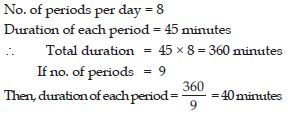##### Question 35:

Take a sheet of paper. Fold it as shown in the figure. Count the number of parts and the area of part in each case.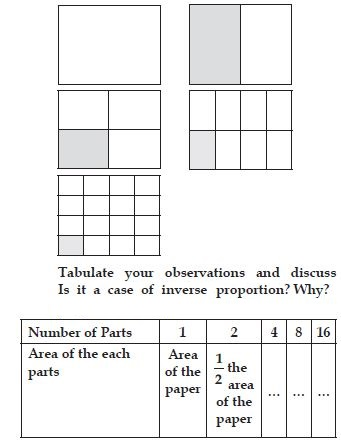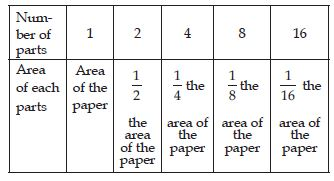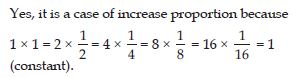##### Question 36:

Take a few containers of different sizes with circular bases. Fill the same amount of water in each container. Note the diameter of each container and the respective height which the water level stands. Tabulate your observations. Is it a case of inverse proportion?